Home | | Transforms and Partial Differential Equations | Partial Differential Equations

# Partial Differential Equations

1 Introduction 2 Formation Of Partial Differntial Equations 3 Solutions Of Partial Differential Equations 4 LagrangeŌĆÖs Linear Equations 5 Partial Differential Equations Of Higher Order With Constant Co-Effecients 6 Non-Homogenous Linear Equations

PARTIAL DIFFERENTIAL EQUATIONS

This unit covers topics that explain the formation of partial differential equations and the solutions of special types of partial differential equations.

1 INTRODUCTION

2 FORMATION OF PARTIAL DIFFERNTIAL EQUATIONS

3 SOLUTIONS OF PARTIAL DIFFERENTIAL EQUATIONS

4 LAGRANGEŌĆÖS LINEAR EQUATIONS

5 PARTIAL DIFFERENTIAL EQUATIONS OF HIGHER ORDER WITH CONSTANT CO-EFFECIENTS

6 NON-HOMOGENOUS LINEAR EQUATIONS

1 INTRODUCTION

A partial differential equation is one which involves one or more partial derivatives. The order of the highest derivative is called the order of the equation. A partial differential equation contains more than one independent variable. But, here we shall consider partial differential only equation two independent variables x and y so that z = f(x,y). We shall denoteA partial differential equation is linear if it is of the first degree in the dependent variable and its partial derivatives. If each term of such an equation contains either the dependent variable or one of its derivatives, the equation is said to be homogeneous, otherwise it is non homogeneous.

2 Formation of Partial Differential Equations

Partial differential equations can be obtained by the elimination of arbitrary constants or by the elimination of arbitrary functions.

By the elimination of arbitrary constants

Let us consider the function

f( x, y, z, a, b  ) = 0  -------------  (1)

where a & b are arbitrary constants

Differentiating equation (1) partially      w.r.t x & y, we getEliminating a and b from equations (1), (2) and (3), we get a partial differential equation of the first order of the form f (x,y,z, p, q) = 0

Example 1

Eliminate      the arbitrary constants a & b        from  z = ax + by + ab

Consider      z  = ax + by + ab     ____________ (1)

Differentiating        (1) partially w.r.t     x & y, we get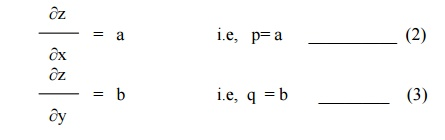Using (2)      & (3)  in (1), we get

z        = px +qy+ pq

which is the required partial differential equation.

Example 2

Form the partial differential equation by eliminating the arbitrary constants a and b from

z = ( x2 +a2 ) ( y2 + b 2)

Given z = ( x2 +a2 ) ( y2 + b2)         ŌĆ”ŌĆ”..(1)

Differentiating        (1) partially w.r.t     x & y , we get

p        = 2x   (y2 + b2 )

q        = 2y   (x  + a  )

Substituting the values of p and q in (1), we get

4xyz = pq

which is the required partial differential equation.

Example 3

Find the partial differential equation of the family of spheres of radius one whose centre lie in the xy - plane.

The equation of the sphere is given by

( x ŌĆōa )2 + ( y- b) 2 +  z2  = 1    _____________ (1)

Differentiating (1) partially w.r.t x & y , we get

2        (x-a ) + 2 zp   =       0

2 ( y-b ) + 2 zq  =    0

From these equations we obtain

x-a = -zp _________ (2)

y -b = -zq _________ (3)

Using (2) and (3) in (1),  we get

z2p2 + z2q2 + z 2    = 1

or  z2 ( p2  + q2  + 1) = 1

Example 4

Eliminate the arbitrary constants a, b & c  fromand form the partial differential equation.

The given equation isor  -zp + xzr + p2x = 0

By the elimination of arbitrary functions

Let   u and v   be   any two   functions arbitrary function. This relation can be expressed as

u = f(v)  ______________ (1)

Differentiating  (1)  partially w.r.t    x &  y and eliminating    the arbitrary functions from these  relations, we get  a partial differential equation  of the first  order of the form

f(x, y, z, p, q )  = 0.

Example 5

Obtain the partial differential equation  by eliminating ŌĆ×fŌĆ×from  z = ( x+y ) f ( x2 -  y2 )

Let us now consider the equation

z = (x+y ) f(x2- y2) _____________ (1)

Differentiating (1) partially w.r.t x & y , we get

p  = ( x + y ) f ' ( x2 -  y2 ) . 2x  +  f ( x2 -  y2 )

q  =  ( x + y ) f ' ( x2 -  y2 ) . (-2y)     + f ( x2 -  y2 )i.e, py - yf( x2 - y2 ) = -qx +xf ( x2 - y2 )

i.e, py +qx   = ( x+y ) f ( x2 -  y2 )

Therefore, we have by(1),  py +qx  = z

Example 6

Form the partial differential equation by eliminating the arbitrary function f

from

z = ey f (x + y)

Consider  z  = ey f ( x +y )  ___________  ( 1)

Differentiating  (1) partially  w .r. t  x & y, we get

p     = ey f ' (x  + y)

q     = ey f '(x  + y) + f(x + y). ey

Hence, we have

q = p + z

Example 7Exercises:

1. 1. Form the partial differential equation by eliminating the arbitrary constants ŌĆ×aŌĆ¤ & ŌĆ×bŌĆ¤ from the following equations.2.                 Find the PDE of the family of spheres of radius 1 having their centres lie on the xy plane{Hint: (x ŌĆōa)2 + (y ŌĆōb)2 + z2 = 1}

3.                 Find the PDE of all spheres whose centre lie on the (i) z axis (ii) x-axis

4.                 Form the partial differential equations by eliminating the arbitrary functions in the following cases.

(i)                z = f (x + y)

(ii)             z = f (x2 ŌĆōy2

(iii)           z = f (x2 + y2 + z2)

(iv)           f(xyz, x + y + z) = 0

(v)             F (xy + z2, x + y + z) = 0

(vi)           z = f (x + iy) +f (x ŌĆōiy)

(vii)        z = f(x3 + 2y) +g(x3 ŌĆō2y)

SOLUTIONS OF A PARTIAL DIFFERENTIAL EQUATION

A solution or integral of a partial differential equation is a relation connecting the dependent and the independent variables which satisfies the given differential equation. A partial differential equation can result both from elimination of arbitrary constants and from elimination of arbitrary functions as explained in section 1.2. But, there is a basic difference in the two forms of solutions. A solution containing as many arbitrary constants as there are independent variables is called a complete integral. Here, the partial differential equations contain only two independent variables so that the complete integral will include two constants.A solution obtained by giving particular values to the arbitrary constants in a complete integral is called a particular integral.

Singular Integral

Let  f (x,y,z,p,q) = 0 ----------   (1)

be the partial differential equation whose complete integral is

f (x,y,z,a,b) = 0-----------          (2)

where          ŌĆ×aŌĆ¤   and   ŌĆ×bŌĆ¤   are   arbitrary   constants.

Differentiating (2) partially w.r.t. a  and b, we obtainThe eliminant of ŌĆ×aŌĆ¤ and ŌĆ×bŌĆ¤ from the equations (2), (3) and (4), when it exists, is called the singular integral of (1).

General Integral

In the complete integral (2), put b = F(a), we get

f (x,y,z,a, F(a) ) = 0       ----------       (5)

Differentiating (2), partially w.r.t.a,  we getThe eliminant of ŌĆ×aŌĆ¤ between (5) and (6), if it exists, is called the general integral of (1).

SOLUTION OF STANDARD TYPES OF FIRST ORDER PARTIAL

DIFFERENTIAL EQUATIONS.

The first order partial differential equation can be written as

f(x,y,z, p,q) = 0,

where p = ┬Čz/┬Čx and q = ┬Čz / ┬Čy. In this section, we shall solve some standard forms of equations by special methods.

Standard I : f (p,q) = 0. i.e, equations containing p and q only.

Suppose that z = ax + by +c is a solution of the equation f(p,q) = 0, where f (a,b)

= 0.

Solving this for b, we get b = F (a).

 Hence the complete integral is z = ax + F(a) y +c------------ (1)

Now, the singular integral is obtained by eliminating a & c between

z = ax + y F(a) + c 0 = x + y F'(a)

0 = 1.

The last equation being absurd, the singular integral does not exist in this case.

To obtain the general integral, let us take c = F (a).

Then, z = ax + F(a) y + F  (a)  -------------- (2)

Differentiating (2) partially w.r.t. a, we get

0 = x + F'(a). y + F '(a)  --------------- (3)

EliminatingŌĆ¤betweenŌĆ×a  (2)   and   (3),   we   get   the   gene

Example 8

Solve pq = 2

The given equation is of the form  f (p,q) = 0

The solution is  z = ax + by +c, where ab = 2.

Solving,      b =  2/b

The complete integral is

Z =  ax  + 2/a y + c        ---------- (1)

Differentiating      (1)   partially   w.r.t   ŌĆ×cŌĆ¤,   we

0 = 1,

which is absurd. Hence, there is no singular integral.

To find the general integral, put c = ’üå (a) in (1), we get

Z = ax + 2/a y + F (a)

Differentiating      partially   w.r.t   ŌĆ×aŌĆ¤,   we   get

0 = x ŌĆō 2/ a2 y + F ŌĆś(a)

Eliminating ŌĆ×aŌĆ¤ between these equations gives the general integral.

Example 9

Solve pq + p +q = 0

The given equation is of the form f (p,q) = 0.

The solution is  z = ax + by +c, where ab + a + b = 0.

Solving, we getDifferentiating (1) partially w.r.t. ŌĆ×cŌĆ¤, we get

0 = 1.

The above equation being absurd, there is no singular integral for the given partial differential equation.

To find the general integral, put c = F (a) in (1), we haveDifferentiating (2) partially w.r.t a, we get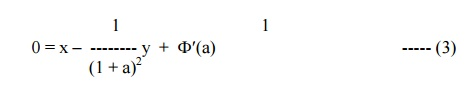Example 10

Solve p2 + q2 = npq

The solution of this equation is  z = ax + by + c, where a2 + b2 = nab.

Solving, we getDifferentiating (1) partially w.r.t c, we get  0 = 1, which is absurd. Therefore, there is no singular integral for the given equation.

To find the general Integral,    put C = F (a), we getThe eliminant of ŌĆ×aŌĆ¤ between these equations gives the general integral

Standard II : Equations of the form f (x,p,q) = 0, f (y,p,q) = 0 and f (z,p,q) = 0. i.e, one of the variables x,y,z occurs explicitly.

(i)                Let us consider the equation f (x,p,q) = 0.

Since z is a function of x and y, we have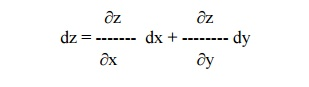or        dz = pdx + qdy

Assume that q = a.

Then the given equation takes the form f (x, p,a ) = 0

Solving, we get   p = F(x,a).

Therefore,        dz = F(x,a) dx + a dy.

(ii) Let us consider the equation f(y,p,q) = 0. Assume that p = a.

Then the equation becomes f (y,a, q) = 0 Solving, we get q = F (y,a).

Therefore, dz = adx + F(y,a) dy.

Integrating, z = ax + ├▓F(y,a) dy + b, which is a complete  Integral.

(iii) Let us consider the equation f(z, p, q) = 0.

Assume that q = ap.

Then the equation becomes f (z, p, ap) = 0

Solving, we get p = F(z,a). Hence dz = F(z,a) dx + a F(z, a) dy.Example 11

Solve q = xp + p2

Given q = xp + p2   -------------(1)

This is of the form f (x,p,q) = 0.

Put q = a in (1), we get

a = xp + p2

i.e, p2 + xp ŌĆōa = 0.

Therefore,Example 12

Solve q = yp2

This is of the form f (y,p,q) = 0

Then, put p = a.

Therfore, the given equation becomes q = a2y.

Since dz = pdx + qdy, we have

dz = adx + a2y dy

Integrating, we get z = ax + (a2y2/2) + b

Example 13

Solve 9 (p2z + q2) = 4

This is of the form f (z,p,q) = 0

Then, putting       q = ap, the given  equation becomes

9 (p2z + a2p2) = 4or      (z + a2)3/2 = x + ay + b.

Standard III : f1(x,p) = f2 (y,q). ie, equations in which ŌĆśzŌĆÖ is absent and the variables are separable.

Let us assume as a trivial solution that

f(x,p) = g(y,q) = a (say).

Solving for p and q, we get p = F(x,a) and q = G(y,a).Hence dz = pdx + qdy = F(x,a) dx + G(y,a) dy

Therefore, z = ├▓F(x,a) dx + ├▓G(y,a) dy + b , which is the complete integral of the given equation containing two constants a and b. The singular and general integrals are found in the usual way.

Example 14

Solve pq = xy

The given equation can be written asExample 15

Solve p2 + q2 = x2 + y2

The given equation can be written as p2 ŌĆōx2 = y2 ŌĆōq2 = a2 (say)

p2 ŌĆōx2 = aImplies p = ├¢(a2 + x2)

andy2 ŌĆōq2 = aImplies q = ├¢(y2 ŌĆōa2)

But   dz = pdx + qdyStandard IV (ClairautŌĆÖs) form

Equation of the type z = px + qy + f (p,q) ------(1) is   known   as   ClairautŌĆ¤s

Differentiating (1) partially w.r.t x and y, we get p = a and q = b.

Therefore, the complete integral is given by

z = ax + by + f (a,b).

Example 16

Solve z = px + qy +pq

The given equation is   in   ClairautŌĆ¤s.   form

Putting p = a and q = b, we have

z = ax + by + ab                                         -------- (1)

which is the complete integral.

To find the singular integral, differentiating (1) partially w.r.t a and b, we get

0 = x + b

0 = y + a

Therefore we have, a = -y and b= -x.

Substituting the values of a & b in (1), we get

z = -xy ŌĆōxy + xy

or      z + xy = 0,  which is the singular integral.

To get the general integral, put b = F(a) in (1).

Then z = ax + F(a)y + a F(a)                              ---------- (2)

Differentiating (2) partially w.r.t a, we have

0 = x + F'(a) y + aF'(a) + F(a)                  ---------- (3)

Eliminating ŌĆ×aŌĆ¤ between (2) and (3), we get the general integral.

Example 17

Find the complete and singular solutions of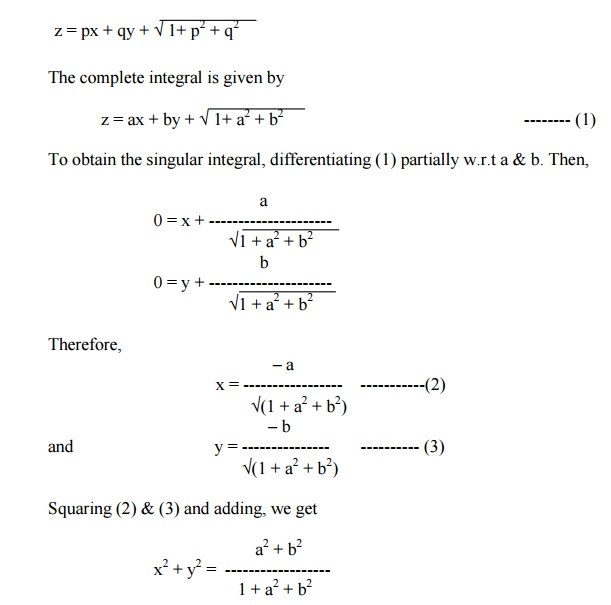Exercises

Solve the following Equations

1.     pq = k

2.     p + q = pq

3.     ├¢p +├¢q = x

4.     p = y2q2

5.     z = p2 + q2

6.     p + q = x + y

7.     p2z2  + q2 = 1

8.     z = px + qy - 2├¢pq

9.     {z ŌĆō(px + qy)}2 = c2 + p2 + q2

10.                        z = px + qy + p2q2

EQUATIONS REDUCIBLE TO THE STANDARD FORMS

Sometimes, it is possible to have non ŌĆōlinear partial differential equations of the first order which do not belong to any of the four standard forms discussed earlier. By changing the variables suitably, we will reduce them into any one of the four standard forms.

Type (i) : Equations of the form F(xm p, ynq) = 0 (or) F (z, xmp, ynq) = 0.

Case(i) : If m ┬╣1 and n ┬╣1, then put x1-m = X and y1-n = Y.Hence, the given equation takes the form F(P,Q) = 0 (or) F(z,P,Q) = 0.

Case(ii) : If m = 1 and n = 1, then put log x = X and log y = Y.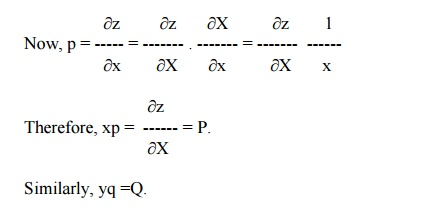Example 18

Solve x4p2 + y2zq = 2z2

The given equation can be expressed as

(x2p)2 + (y2q)z = 2z2

Here m = 2, n = 2

Put X = x1-m = x -1  and  Y = y 1-n = y -1.

We have xmp = (1-m) P  and ynq = (1-n)Q

i.e, x2p = -P and  y2q = -Q.

Hence the given equation becomes

P2 ŌĆōQz = 2z----------(1)

This equation is of the form f (z,P,Q) = 0.

Let us take Q = aP.

Then equation (1) reduces to

P2 ŌĆōaPz =2z2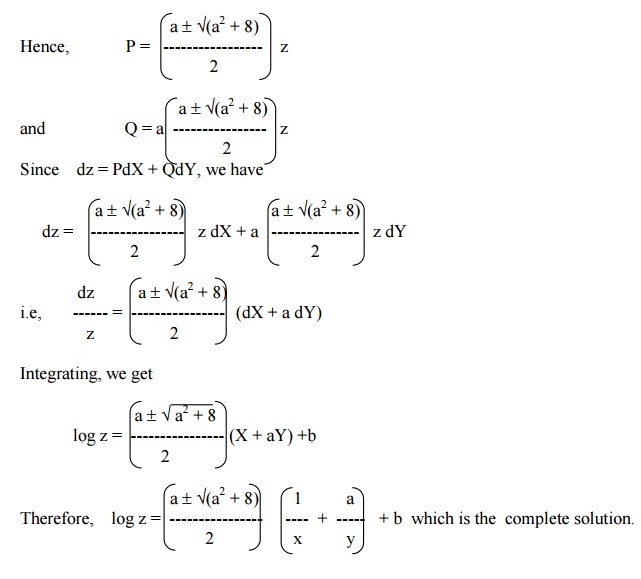Example 19

Solve x2p2 + y2q2  = z2

The given equation can be written as

(xp)2 + (yq)2  = z2

Here m = 1, n = 1.

Put X = log x  and Y = log y.

Then xp = P  and yq = Q.

Hence the given equation becomes

P2 + Q2  = z2   ------------- (1)

This equation is of the form F(z,P,Q) = 0.

Therefore, let us assume that Q = aP.

Now, equation (1) becomes,

P2 + a2 P2 = z2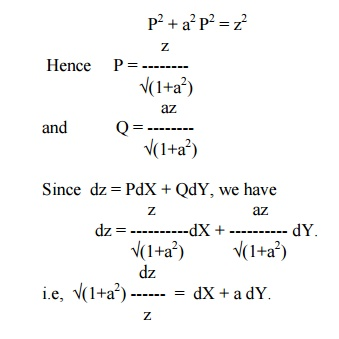Integrating, we get

├¢(1+a2) log z = X + aY + b.

Therefore, ├¢(1+a2) log z = logx + alogy + b, which is the complete solution.

Type (ii) : Equations of the form F(zkp, zkq) = 0 (or) F(x, zkp) = G(y,zkq).

Case (i) : If k ┬╣-1, put Z = zk+1,Example 20

Solve z4q2 ŌĆōz2p = 1

The given equation can also be written as

(z2q)2 ŌĆō(z2p) =1

Here k = 2. Putting Z = z k+1 = z3, we geti.e,   Q2 ŌĆō3P ŌĆō9 = 0,

which is of the form F(P,Q) = 0.

Hence its solution is Z = ax + by + c, where b2 ŌĆō3a ŌĆō9 = 0.

Solving for b,  b = ┬▒ ├¢(3a +9)

Hence the complete solution is

Z = ax  + ├¢(3a +9) . y + c

or      z3 = ax  + ├¢(3a +9) y + c4 LagrangeŌĆÖs   Linear   Equation

Equations of the form Pp + Qq  = R ________ (1), where P, Q and R are functions   of x,   y,   z,   are   known   as   Lagrang solve this equation, let us consider the equations u = a  and v = b, where a, b are arbitrary constants and u, v are functions of x, y, z.Equations (5) represent a pair of simultaneous equations which are of the first order and of first degree.Therefore, the two solutions of (5) are u = a and v = b. Thus, f( u, v ) = 0 is the required solution of (1).

Note :

To solve the LagrangeŌĆ¤s equation,we have to form the subsidiary or auxiliary  equationswhich can be solved either by the method of grouping or by the method of multipliers.

Example 21

Find the  general solution of px + qy = z.

Here, the subsidiary equations areIntegrating, log x = log y + log c1

or x = c1 y i.e, c1 = x / y

From the last two ratios,Integrating, log y = log z  + log c2

or  y = c2 z

i.e,  c2 = y / z

Hence the required  general solution is

╬”(   x/y,=  0,y/z)where ╬” is   arbitrary

Example 22

Solve  p tan x + q tan y  = tan z

The subsidiary equations areHence the required  general solution iswhere ╬” is   arbitrary

Example 23

Solve (y-z) p + (z-x) q  = x-y

Here the subsidiary equations areExample 24

Find the general solution of  (mz  - ny) p  + (nx- lz)q = ly - mx.Exercises

Solve the following equations

1.     px2 + qy2 = z2

2.     pyz + qzx = xy

3.     xp ŌĆōyq = y2 ŌĆōx2

4.     y2zp + x2zq = y2

5.     z (x ŌĆōy) = px2 ŌĆōqy2

6.     (a ŌĆōx) p + (b ŌĆōy) q = c ŌĆōz

7.  (y2z p) /x  + xzq = y2

8.     (y2 + z2) p ŌĆōxyq + xz = 0

9.     x2p + y2q = (x + y) z

10.                      p ŌĆōq = log (x+y)

11.                      (xz + yz)p + (xz ŌĆōyz)q = x2 + y2

12.                      (y ŌĆōz)p ŌĆō(2x + y)q = 2x + z

5 PARTIAL DIFFERENTIAL EQUATIONS OF HIGHER ORDER WITH CONSTANT COEFFICIENTS.

Homogeneous Linear Equations with constant Coefficients.

A homogeneous linear partial differential equation of the nth order is of the formhomogeneous because all its terms contain derivatives of the same order.

Equation (1) can be expressed asAs in the case of ordinary linear equations with constant coefficients the complete solution of (1) consists of two parts, namely, the complementary function and the particular integral.

The complementary function is the complete solution of f (D,D') z = 0-------(3), which must contain n arbitrary functions as the degree of the polynomial f(D,D'). The particular integral is the particular solution of equation (2).

Finding the complementary function

Let us now consider the equation f(D,D') z = F (x,y)

The auxiliary equation of (3) is obtained by replacing D by m and D' by 1.Solving equation (4) for ŌĆ×mŌĆ¤, we get ŌĆ×nŌĆ¤ roots. Depending upon the nature of the roots, the Complementary function is written as given below:Finding the particular IntegralExpand [f (D,D')]-1 in ascending powers of D or D' and operate on xm yn term by term.

Case (iv) : When F(x,y) is any function of x and y.into partial fractions considering f (D,D') as a function of D alone.

Then operate each partial fraction on F(x,y)  in such a way thatwhere c is replaced by y+mx after integration

Example 26

Solve(D3 ŌĆō3D2D' + 4D'3) z = ex+2y

The auxillary equation is m=m3 ŌĆō3m2 + 4 = 0

The roots are m = -1,2,2

Therefore the C.F is f1(y-x) + f2 (y+ 2x) + xf3 (y+2x).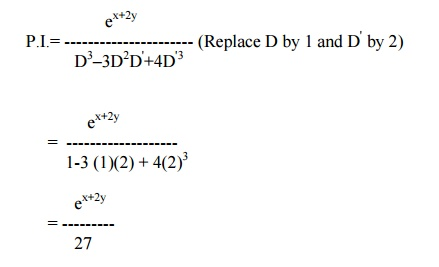Hence, the solution is z  = C.F. + P.IExample 27

Solve (D2 ŌĆō4DD' +4 D' 2) z  = cos (x ŌĆō2y)

The auxiliary equation is m2 ŌĆō4m + 4 = 0

Solving, we get m = 2,2.

Therefore the        C.F is f1(y+2x) + xf2(y+2x).Example 28

Solve (D2 ŌĆō2DD') z = x3y + e5x

The auxiliary equation is m2 ŌĆō2m = 0.

Solving, we get     m = 0,2.

Hence the    C.F    is       f1 (y) + f2 (y+2x).Example 29The auxiliary equation is m2 + m ŌĆō6 = 0.

Therefore,   m = ŌĆō3, 2.

Hence the C.F is   f1(y-3x) + f2(y + 2x).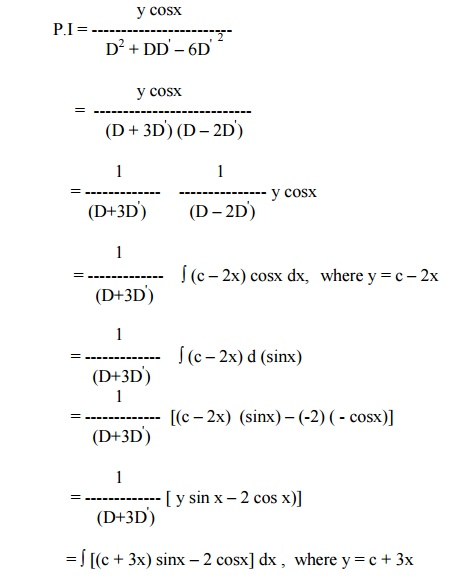=   ├▓(c + 3x) d(ŌĆōcosx) ŌĆō2├▓cosx  dx

= (c + 3x) (ŌĆōcosx) ŌĆō(3) ( - sinx) ŌĆō2 sinx

=   ŌĆōy cosx + sinx

Hence the complete solution is

z = f1(y ŌĆō3x) + f2(y + 2x) ŌĆōy cosx + sinx

Example 30

Solve r ŌĆō4s + 4t = e 2x +yi.e, (D2 ŌĆō4DD' + 4D' 2  ) z = e2x + y

The auxiliary equation is m2 ŌĆō4m + 4 = 0.

Therefore, m = 2,2

Hence the C.F is f1(y + 2x) + x f2(y + 2x).Since D2 ŌĆō4DD'+4D'2 = 0 for D = 2  and D' = 1, we have to apply the general rule.6 Non ŌĆōHomogeneous Linear Equations

Let us consider the partial differential equation

f (D,D') z = F (x,y)------- (1)

If f (D,D') is not homogeneous, then (1) is a nonŌĆōhomogeneous linear partial differential equation. Here also, the complete solution = C.F + P.I.

The methods for finding the Particular Integrals are the same as those for homogeneous linear equations.

But for finding the C.F, we have to factorize f (D,D') into factors of the form D ŌĆōmD' ŌĆōc.

Consider now the equation

(D ŌĆōmD' ŌĆōc) z = 0 -----------  (2).

This equation can be expressed as

p ŌĆōmq = cz ---------(3),

which is in Lagrangian form.

The subsidiary equations areThe solutions of (4) are y + mx = a and z = becx.

Taking b =  f (a), we get z = ecx f (y+mx) as the solution of (2).

Note:

1.  If   (D-m1D' ŌĆōC1) (D ŌĆōm2D'-C2)   ŌĆ”ŌĆ” ŌĆōm(Dn'-Cn) z = 0 is the partial

differential equation, then its complete solution is

z = ec1x f1(y +m1x) + ec2x f2(y+m2x) + . . . . . + ecnx fn(y+mnx)

2.  In the case of repeated factors, the equation (D-mD' ŌĆōC)nz = 0 has a complete

solution z = ecx f1(y +mx) + x ecx f2(y+mx) + . . . . . +x n-1 ecx fn(y+mx).

Example 31

Solve (D-D'-1) (D-D' ŌĆō2)z = e 2x ŌĆōy

Here  m1 = 1, m2 = 1, c1 = 1, c2 = 2.

Therefore, the C.F is ex f1 (y+x) + e2x f2 (y+x).Example 32

Solve (D2 ŌĆōDD' + D' ŌĆō1) z = cos (x + 2y)

The given equation can be rewritten as

(D-D'+1) (D-1) z = cos (x + 2y)

Here m1 = 1, m2 = 0, c1 = -1, c2 = 1.

Therefore, the C.F = eŌĆōx f1(y+x) + ex f2 (y)Example 33

Solve [(D + D'ŌĆō1) (D + 2D' ŌĆō3)] z = ex+2y + 4 + 3x +6y

Here m1 = ŌĆō1, m2 = ŌĆō2 , c1 = 1, c2 = 3.

Hence the C.F is z = ex f1(y ŌĆōx) + e3x f2(y ŌĆō2x).It is the complete solution.

Exercises

(a) Solve the following homogeneous Equations.6. (D2 + 4DD' ŌĆō5D'2) z = 3e2x-y + sin (x ŌĆō2y)

7. (D2 ŌĆōDD' ŌĆō30D'2) z  = xy + e6x+y

8. (D2 ŌĆō4D' 2) z = cos2x. cos3y

9. (D2 ŌĆōDD'  ŌĆō2D'2) z = (y ŌĆō1)ex

10.4r + 12s + 9t =  e3x ŌĆō2y

(b)Solve the following non ŌĆōhomogeneous equations.

1.     (2DD' + D' 2 ŌĆō3D') z = 3 cos(3x ŌĆō2y)

2.     (D2 + DD' + D' ŌĆō1) z = e-x

3.     r ŌĆōs + p = x2 + y2

4.     (D2 ŌĆō2DD' + D'2 ŌĆō3D + 3D' + 2)z = (e3x + 2e-2y)2

(D2 ŌĆōD'2 ŌĆō3D + 3D') z = xy + 7.

Study Material, Lecturing Notes, Assignment, Reference, Wiki description explanation, brief detail
Mathematics (maths) : Partial Differential Equations : Partial Differential Equations |